How to Apply Sine Rule, Cosine Rule and Tangent Rule in Trigonometry

Sine Rule

In trigonometry, the sine law, law of sines, sine rule, or sine formula is an rational equation that relates to the lengths of the sides of a triangle (any shape or kind) to the sines of its angles.

According to the sine rule,

a / sin(A) = b / sin(B) = c / sin(C)

where a, b, and c are the lengths of the sides of a triangle, and A, B, and C are the opposite angles.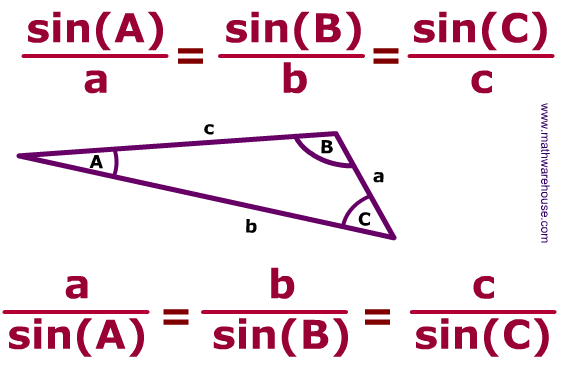Application of Sine Rule

• Sine rule can be used to find the unknown side or length of a triangle.
• Sine rule can also be used to find the unknown angle of a triangle.

To find the unknown side or length of a triangle, three essential parameters must be provided and these parameters are:

• The angle opposite to the unknown side or length of the triangle.
• The length of a side of the triangle (b).
• The angle opposite to the length of side (b) of the triangle.

Let’s take for example we have a triangle and we want to determine the unknown side of this triangle, when the opposite angle is given, another length of the side of the triangle and its opposite angle is also given. Like the image below.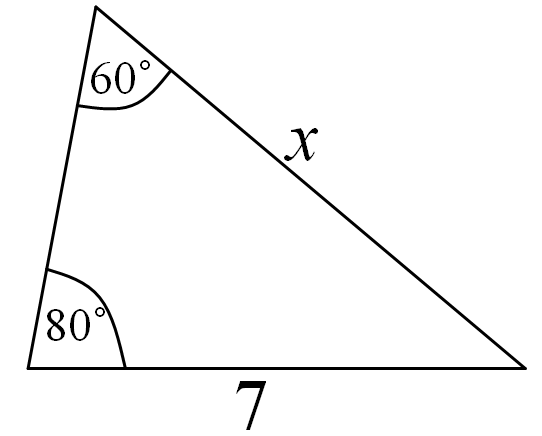From the image above, one can see that;
The angle opposite to the unknown length of the side of the triangle is 80°. (A)
The length of the side of the triangle given is 7. (b)
The angle opposite to the length of the side of the triangle given is 60° (B)
The unknown length of the side of the triangle is x. (a)

Applying the sine rule to find the value of x:

a / sin(A) = b / sin(B)

x / sin(80°) = 7 / sin(60°)
x / 0.9848 = 7 / 0.8660

Applying cross multiplication

x (0.8660) = 7 (0.9848)
x (0.8660) = 6.8936

Dividing both sides by 0.8660

x = 6.8936 / 0.8660
x = 7.96

Therefore, the unknown length of the side of the triangle, x is 7.96.

How to Calculate and Solve for the Height and Volume of a Conical Frustum | The Calculator Encyclopedia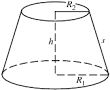The image above is a conical frustum.

To compute the volume of a conical frustum, three essential parameters are needed and this parameters are radius of the lower base (R), radius of the upper base (r) and height (h).

The formula for calculating the volume of a conical frustum:

V = πh(R² + Rr + r²)3

Where;
V = Volume of the conical frustum
R = Radius of the lower base
r = Radius of the upper base
h = Height of the conical frustum

Let’s solve an example;
Find the volume of the conical frustum when the lower base is 5 cm with an upper base of 9 cm and a height of 11 cm.

This implies that;
R = Radius of the lower base = 5 cm
r = Radius of the upper base = 9 cm
h = Height of the conical frustum = 11 cm

V = π(11)((5)² + (5)(9) + (9)²)3
V = (34.557)((25) + (45) + (81))3
V = (34.557)(151)3
V = 5218.1853
V = 1739.39

Therefore, the volume of the conical frustum is 1739.39 cm3.

How to Calculate and Solve for the Height and Volume of a Square Pyramid Frustum | Nickzom Calculator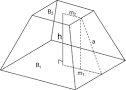The image above is a square pyramid frustum.

To compute the volume of a square pyramid frustum, three essential parameters are needed and this parameters are base side length (a), top side length (b) and height (h).

The formula for calculating the volume of a square pyramid frustum:

V = h(a² + ab + b²)3

Where;
V = Volume of a square pyramid frustum
a = Base side length of the square pyramid frustum
b = Top side length of the square pyramid frustum
h = Height of the square pyramid frustum

Let’s solve an example
Find the volume of a square pyramid frustum when the base side length is 10 cm with a top side length of 12 cm and a height of 20 cm.

This implies that;
a = Base side length of the square pyramid frustum = 10 cm
b = Top side length of the square pyramid frustum = 12 cm
h = Height of the square pyramid frustum = 20 cm

V = h(a² + ab + b²)3
V = 20(10² + (10 x 12) + 12²)3
V = 20(100 + (120) + 144)3
V = 20(364)3
V = 72803
V = 2426.6

Therefore, the volume of the square pyramid frustum is 2426.6 cm3.

Calculating the Height of a Square Pyramid Frustum when the Volume, Top Side Length and Base Side Length of the Square Pyramid Frustum is Given.

h = 3V / (a2 + ab + b2)

Where;
h = Height of the square pyramid frustum
V = Volume of the square pyramid frustum
a = Base side length of the square pyramid frustum
b = Top side length of the square pyramid frustum

Let’s solve an example;
Find the height of a square pyramid frustum when the volume is 32 cm3, base side length is 14 cm and top side length is 18 cm.

This implies that;
V = Volume of the square pyramid frustum = 32 cm3
a = Base side length of the square pyramid frustum = 14 cm
b = Top side length of the square pyramid frustum = 18 cm

h = 3V / (a2 + ab + b2)
h = 32 x 3 / (142 + (14 x 18) + 182)
h = 96 / (196 + 252 + 324)
h = 96 / 772
h = 0.124

Therefore, the height of the square pyramid frustum is 0.124 cm.

How to Calculate and Solve for the Height, Base Edge and Area of a Square Pyramid | Nickzom Calculator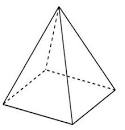The image above is a square pyramid.

To compute the area of a square pyramid, two essential parameters are needed and this parameters are base edge (a) and height (h).

The formula for calculating the area of a square pyramid:

A = a² + 2a√(( / 4) + h²)

Where;
A = Area of a square pyramid
a = Base edge of the square pyramid
h = Height of the square pyramid

Let’s solve for an example;
Find the area of a square pyramid when the base edge (a) given is 8 cm with a height (h) of 12 cm.

This implies that;
a = Base edge of the square pyramid = 8 cm
h = Height of the square pyramid = 12 cm

A = a² + 2a√(( / 4) + h²)
A = 8² + 2 x 8√(( / 4) + 12²)
A = 64 + 16√((64 / 4) + 144)
A = 64 + 16√(16 + 144)
A = 64 + (16)(√160)
A = 64 + (16)(12.65)
A = 64 + 202.3857
A = 266.386

Therefore, the area of the square pyramid is 266.386 cm2.

How to Calculate and Solve for the Area of an Ellipsoid | The Calculator Encyclopedia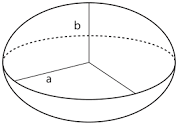The image above is an ellipsoid.

To compute the area of an ellipsoid, three essential parameters are needed and this parameters are axis (a)axis (b) and axis (c).

The formula for calculating the area of an ellipsoid:

A = 4π((ab)1.6 + (ac)1.6 + (bc)1.63)1 / 1.6

Where;
A = Area of the ellipsoid
a = Axis of the ellipsoid
b = Axis of the ellipsoid
c = Axis of the ellipsoid

Let’s solve an example;
Find the the area of an ellipsoid when the axis (a) of the ellipsoid is 12 cm, axis (b) of the ellipsoid is 6 cm and axis (c) of the ellipsoid is 2 cm.

This implies that;
a = Axis of the ellipsoid = 12 cm
b = Axis of the ellipsoid = 6 cm
c = Axis of the ellipsoid = 2 cm

A = 4π((ab)1.6 + (ac)1.6 + (bc)1.63)1 / 1.6
A = 4π(((12)(6))1.6 + ((12)(2))1.6 + ((6)(2))1.63)1 / 1.6
A = 4π(((72)1.6 + (24)1.6 + (12)1.6)3)1 / 1.6
A = 4π(((936.98) + (161.56) + (53.29))3)1 / 1.6
A = 4π((1151.84)3)1 / 1.6
A = 4π(383.946)1 / 1.6
A = 4π(383.946)0.625
A = 4π(41.2258)
A = (12.566)(41.2258)
A = 518.059

Therefore, the area of the ellipsoid is 518.059 cm2.

How to Calculate and Solve for the Axis and Volume of an Ellipsoid | The Calculator EncyclopediaThe image above is an ellipsoid.

To compute the volume of an ellipsoid, three essential parameters are needed and this parameters are axis (a)axis (b) and axis (c).

The formula for calculating the volume of an ellipsoid:

V = 4πabc3

Where;

V = Volume of the ellipsoid
a = Axis of the ellipsoid
b = Axis of the ellipsoid
c = Axis of the ellipsoid

Let’s solve an example;
Given that the axis of the ellipsoid (a) is 9 cm and axis of the ellipsoid (b) is 11 cm with the axis (c) of 14 cm. Find the volume of the ellipsoid?

This implies that;
a = Axis of the ellipsoid = 9 cm
b = Axis of the ellipsoid = 11 cm
c = Axis of the ellipsoid = 14 cm

V = 4πabc3
V = 4π(9 x 11 x 14)3
V = 4π(1386)3
V = (12.56)(1386) / 3
V = (17416.98967) / 3
V = 5805.66

Therefore, the volume of the ellipsoid is 5805.66 cm3.

Calculating the Axis (a) of an Ellipsoid using the Volume of the Ellipsoid, Axis (b) of the Ellipsoid and Axis (c) of the Ellipsoid.

a = V3 / 4πbc

Where;
a = Axis of the ellipsoid
V = Volume of the ellipsoid
b = Axis of the ellipsoid
c = Axis of the ellipsoid

Let’s solve an example;
Find the axis (a) of an ellipsoid when the volume of the ellipsoid is 280 cm3 with an axis (b) of 18 cm and axis (c) of 8 cm.

This implies that;
V = Volume of the ellipsoid = 280 cm3
b = Axis of the ellipsoid = 18 cm
c = Axis of the ellipsoid = 8 cm

a = V3 / 4πbc
a = 280 x 3 / (12.566)(18 x 8)
a = 840 / (12.566)(144)
a = 840 / 1809.504
a = 0.46

Therefore, the axis (a) of the ellipsoid is 0.46 cm.

How to Calculate and Solve for the Radius, Height and Surface Area of a Spherical Segment | The Calculator Encyclopedia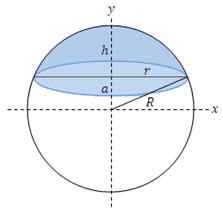The image above is a spherical segment.

To compute the surface area of a spherical segment requires two essential parameters which are the radius of the sphere (R) and the height (h).

The formula for calculating the surface area of the spherical segment:

A = 2πRh

Where;
A = Surface area of the spherical segment
R = Radius of the sphere
h = Height of the spherical segment

Let’s solve an example;
Find the surface area of a spherical segment when the radius of the sphere is 12 cm and the height is 16 cm.

This implies that;
R = Radius of the sphere = 12 cm
h = Height of the spherical segment = 16 cm

A = 2πRh
A = 2π (12 x 16)
A = 2π (192)
A = 6.28 (192)
A = 1206.37

Therefore, the surface area of the spherical segment is 1206.37 cm2.

Calculating the Radius of the Sphere using the Surface Area of the Spherical Segment and the Height.

R = A / 2πh

Where;
R = Radius of the sphere
A = Surface area of the spherical segment
h = Height of the spherical segment

Let’s solve an example;
Find the radius of a sphere with a surface area of 300 cm2 and a height of 12 cm.

This implies that;
A = Surface area of the spherical segment = 300 cm2
h = Height of the spherical segment = 12 cm

R = A / 2πh
R = 300 / 2 x π x 12
R = 300 / 75.41
R = 3.978

Therefore, the radius of the sphere is 3.978 cm.

How to Calculate and Solve for the Volume of a Spherical Segment | Nickzom Calculator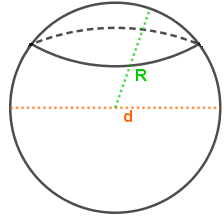The image above is a spherical segment.

To compute the volume of a spherical segment requires three essential parameters which are the radius of the spherical segment base (r1), radius of the spherical segment (r2) and height (h).

The formula for calculating the volume of the spherical segment:

V = πh(3r1² + 3r2² + h²)6

Where;
V = Volume of the spherical cap
r1 = Radius of the spherical segment base
r2 = Radius of the spherical segment base
h = Height of the spherical segment

Let’s solve an example;
Find the volume of a spherical segment when the radius of the spherical segment base (r1) is 7 cm, radius of the spherical segment base (r2) is 9 cm and a height of 20 cm.

This implies that;
r1 = Radius of the spherical segment base = 7 cm
r2 = Radius of the spherical segment base = 9 cm
h = Height of the spherical segment = 20 cm

V = πh(3r1² + 3r2² + h²)6
V = π x 20(3 x 7² + 3 x 9² + 20²)6
V = π x 20(3 x 49 + 3 x 81 + 400)6
V = π x 20(147 + 243 + 400)6
V = π x 20(790)6
V = π x 158006
V = 49643.66
V = 8273.9

Therefore, the volume of the spherical segment is 8273.9 cm3.

How to Calculate the Radius, Height and Curved Surface Area of a Spherical Cap | The Calculator Encyclopedia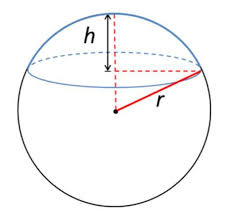The image above is a spherical cap.

To compute the curved surface area of a spherical cap requires two essential parameters which are the radius of the base of the cap (a) and the height (h).

The formula for calculating the curved surface area of the spherical cap:

A = π(a² + h²)

Where;
A = Curved surface area of the spherical cap
a = Radius of the base of the cap
h = Height of the spherical cap

Let’s solve an example;
Find the curved surface area of a spherical cap with radius of the base 7 cm and the height of 13 cm.

This implies that;
a = Radius of the base of the cap = 7 cm
h = Height of the spherical cap = 13 cm

A = π(a² + h²)
A =  π(7² + 13²)
A =  π(49 + 169)
A =  π(218)
A = 684.867

Therefore, the curved surface area of the spherical cap is 684.867 cm².

Calculating the Radius of the base of a Spherical Cap using the Curved Surface Area of the Spherical Cap and the Height.

a = √A – πh2 / π

Where;
A = Curved surface area of the spherical cap
a = Radius of the base of the cap
h = Height of the spherical cap

Let’s solve an example;
Find the radius of the base of a spherical cap when the curved surface area of the spherical cap is 300 cm2 and a height of 7 cm.

This implies that;
A = Curved surface area of the spherical cap = 300 cm2
h = Height of the spherical cap = 7 cm

a = √A – πh2 / π
a = √300 – 3.142 x 72 / π
a = √300 – 3.142 x 49 / π
a = √300 – 153.958 / π
a = √146.042 / π
a = √46.48
a = 6.82

Therefore, the radius of the base of the cap is 6.82 cm.

How to Calculate and Solve for the Volume of a Spherical Cap | Nickzom Calculator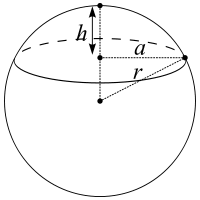The image above is a spherical cap.

To compute the volume of a spherical cap requires two essential parameters which are the radius of the base of the cap (a) and the height (h).

The formula for calculating the volume of the spherical cap:

V = πh(3a² + h²) ⁄ 6

Where;
V = Volume of the spherical cap
a = Radius of the base of the cap
h = Height of the spherical cap

Lets solve an example;
Find the volume of a spherical cap when the radius of the base is 12 cm and the height is 22 cm.

This implies that;
a = Radius of the base of the cap = 12 cm
h = Height of the spherical cap = 22 cm

V = πh(3a² + h²) ⁄ 6
V = 3.142 (22)(3(12)² + 22²) ⁄ 6
V = 3.142 (22)(3(144) + 484) ⁄ 6
V = 3.142 (22)(432 + 484) ⁄ 6
V = 3.142 (22)(916) ⁄ 6
V = 3.142 (20152)6
V = 63309.37 ⁄ 6
V = 10551.56

Therefore, the volume of the spherical cap is 10551.56 cm2.